# Modeling the United States Economy

This example illustrates the use of a vector error-correction (VEC) model as a linear alternative to the Smets-Wouters Dynamic Stochastic General Equilibrium (DSGE) macroeconomic model, and applies many of the techniques of Smets-Wouters to the description of the United States economy.

Moreover, the example highlights many of the more salient features of multiple time series analysis found in Econometrics Toolbox™, including the estimation, Monte Carlo simulation, filtering, forecasting, and impulse response functionality. The example also illustrates how to determine the cointegration rank using the suite of Johansen cointegration tests, as well as the imposition of parameter constraints during estimation.

The Smets-Wouters model (, , ) is a nonlinear system of equations in the form of a DSGE model. DSGE models attempt to explain aggregate economic behavior, such as growth, business cycles, and monetary and fiscal policy effects, using macroeconomic models derived from microeconomic principles.

DSGE models are dynamic; they study how economies change over time. DSGE models are also stochastic; they account for the effects of random shocks including technological changes and price fluctuations.

Because DSGE models start from microeconomic principles of constrained decision-making, rather than relying on historical correlations, they are more difficult to solve and analyze. However, because they are also based on the preferences of economic agents, DSGE models offer a natural benchmark for evaluating the effects of policy change.

In contrast, traditional macroeconometric forecasting models estimate the relationships between variables in different sectors of the economy using historical data, and are generally easier to implement, analyze, and interpret.

This example implements a traditional linear forecasting model. The intent is not to reproduce the results of the original Smets-Wouters DSGE model, nor to interpret its results. Rather, this example illustrates how you can use the features of Econometrics Toolbox to support a workflow similar to that of Smets and Wouters  using the same macroeconomic time series data.

This example uses a cointegrated vector autoregression (VAR) model, also known as a vector error-correction (VEC) model, both of which are supported by various functions in Econometrics Toolbox. This descriptive macroeconomic model offers a nice starting point to examine the impact of various shocks to the United States economy, particularly around the period of the 2008 fiscal crisis. This example uses Johansen cointegration tests and illustrates the estimation, simulation, filtering, and forecasting features of VAR and VEC multivariate time series models in Econometrics Toolbox. In the style of Smets and Wouters, this example models the same 7 response series: output (GDP), prices (GDP deflator), wages, hours worked, interest rates (Federal Funds), consumption, and investment.

Also, to highlight the manipulation and management of quarterly economic time series data, this example uses timetable and datetime datatypes.

### Obtain Economic Data

The file Data_USEconVECModel.mat contains the appropriate economic series stored in two timetables: FRED and CBO. FRED contains the sample to which the model is fit and is from the Federal Reserve Economic Database (FRED)  for periods 1957:Q1 (31-Mar-1957) through 2016:Q4 (31-Dec-2016). CBO is from the Congressional Budget Office (CBO)  and contains 10-year economic projections for a subset of the FRED series. CBO is in the forecast horizon; this example uses observations therein for conditional forecasting and simulation beyond the latest FRED data.

The response series used are:

FRED Series Description ------------ ---------------------------------------------------------------------------- GDP Gross Domestic Product (USD billions, Quarterly) GDPDEF Gross Domestic Product Implicit Price Deflator (Index 2009 = 100, Quarterly) COE Paid Compensation of Employees (USD billions, Quarterly) HOANBS Nonfarm Business Sector Hours of All Persons (Index 2009 = 100, Quarterly) FEDFUNDS Effective Federal Funds Rate (Annualized, Percent, Monthly) PCEC Personal Consumption Expenditures (USD billions, Quarterly) GPDI Gross Private Domestic Investment (USD billions, Quarterly)
load Data_USEconVECModel 

If you have a Datafeed Toolbox™ license, then you can load, synchronize, and merge the latest economic data directly from FRED to better understand a more comprehensive workflow. To download the most recent data directly from FRED, users of Datafeed Toolbox can run the following command:

FRED = Example_USEconData;

FRED returns all data at the beginning of each period. With the exception of Federal Funds, FRED returns quarterly, seasonally adjusted series at the beginning of each quarter. Federal Funds are reported monthly at the beginning of each month. Because the GDP implicit price deflator is in the model, this example uses nominal series throughout.

For consistency with Smets and Wouters, this example synchronizes the FRED data to a quarterly periodicity using an end-of-quarter reporting convention.

For example, FRED reports 2012:Q1 GDP dated 01-Jan-2012 and 2012:M3 Federal Funds dated 01-Mar-2012. When synchronized to a common end-of-quarter periodicity, both series are associated with 31-Mar-2012, the last day of the first quarter of 2012. For any given year YYYY, the data for Q1, Q2, Q3, and Q4 correspond to 31-Mar-YYYY, 30-Jun-YYYY, 30-Sep-YYYY, and 31-Dec-YYYY, respectively.

### Transform Raw Data

Given the raw data from FRED, transform the series as in Smets and Wouters  in which the interest rate (FEDFUNDS) is unchanged while the remaining series that exhibit exponential growth are expressed as 100 times the logarithm of the corresponding reported value. For simplicity, retain the same series names for both raw and transformed data.

Data = FRED; % Assign dates and raw data Data.GDP = 100*log(FRED.GDP); % GDP (output) Data.GDPDEF = 100*log(FRED.GDPDEF); % GDP implicit price deflator Data.COE = 100*log(FRED.COE); % Compensation of employees (wages) Data.HOANBS = 100*log(FRED.HOANBS); % Hours of all persons (hours) Data.PCEC = 100*log(FRED.PCEC); % Personal consumption expenditures (consumption) Data.GPDI = 100*log(FRED.GPDI); % Gross private domestic investment (investment) 

### Display Model Data

To examine the data in the model, plot each series and overlay shaded bands to identify periods of economic recession as determined by the National Bureau of Economic Research (NBER) . The recessionplot function plots recessions, and this example includes recessions in graphical results wherever appropriate. recessionplot arbitrarily sets the middle of the month as the start or end date of a recession.

figure subplot(2,2,1) plot(Data.Time, [Data.GDP, Data.GDPDEF]) recessionplot title('GDP & Price Deflator') ylabel('Logarithm (x100)') h = legend('GDP','GDPDEF','Location','Best'); h.Box = 'off'; subplot(2,2,2); plot(Data.Time, [Data.PCEC Data.GPDI]) recessionplot title('Consumption & Investment') ylabel('Logarithm (x100)') h = legend('PCEC','GPDI','Location','Best'); h.Box = 'off'; subplot(2,2,3) plot(Data.Time, [Data.COE Data.HOANBS]) recessionplot title('Wages & Hours') ylabel('Logarithm (x100)') h = legend('COE','HOANBS','Location','Best'); h.Box = 'off'; subplot(2,2,4) plot(Data.Time, Data.FEDFUNDS) recessionplot title('Federal Funds') ylabel('Percent')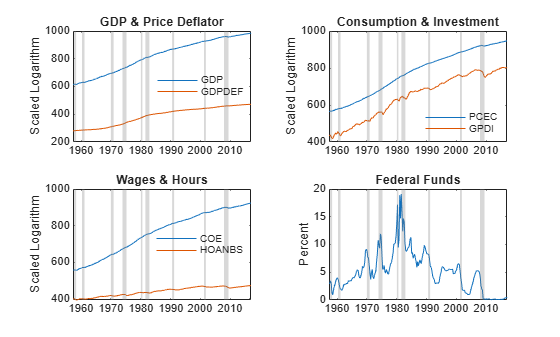### Outline the Estimation Approach

The specification of an appropriate VEC model can incorporate personal experience and familiarity with the data, ad hoc analyses, industry best practice, regulatory mandates, and statistical rigor. Moreover, model specification can be applied to in-sample metrics such as AIC/BIC information criteria, and out-of-sample forecast performance. Although many researchers often include elements of all of these metrics in an iterative fashion, to do so here is beyond the scope of an example.

Given that Smets and Wouters  emphasize out-of-sample forecasting performance, this example does not assess in-sample goodness-of-fit measures. However, Econometrics Toolbox supports such assessments (e.g., see aicbic).

To understand the specification approach in this example, it is useful to observe that VEC model estimation proceeds in two steps:

1. Estimate the cointegrating relations. This is often referred to as the Johansen step. For non-stationary time series, the cointegrating rank (r) is the number of independent linear combinations for whichis stationary, and can be loosely interpreted as the number of long-run equilibrium relations in. In other words, although the component series incan be individually non-stationary, various linear combinations of them are stationary. The number of stationary independent linear combinations is the cointegrating rank, and the corresponding stationary linear combinations are the cointegrating relations.

2. Estimate a VAR model in differences using the cointegrating relations of the first step as predictors. Because the inclusion of predictors adds a regression component to the VAR model, this is often referred to as the VARX step.

The workflow for specifying an appropriate VEC, or cointegrated VAR, model is:

• Determine lag length (P) of the model. Regardless of whether you prefer the VEC(P-1) form in differencesor the VAR(P) form in levels, P represents the number of presample responses needed to initialize the model.

• Determine the cointegrating rank (r).

• Determine a model of cointegration that best captures the deterministic terms of the data.

Collectively, this sequence determines the cointegrating relations and corresponds to the first step of the two-step estimation approach.

Impose additional structure on the parameters of the VARX model:

• Estimate an unrestricted VARX model to serve as a baseline model.

• Determine and impose suitable constraints on the parameters of the VARX model, including the constant, regression coefficients, and autoregressive coefficients.

### Estimate the VEC Model

First specify the lag length (P) of the VEC(P-1) model. This example takes a simple approach and adopts the guidance of Johansen , p. 4, and Juselius , p. 72. Assume that P = 2 lags are sufficient to describe the dynamic interactions between the various series.

The benefit of a low lag order is model simplicity. In particular, each additional lag of an unconstrained VEC model requires the estimation of an additional 7*7 = 49 parameters, so a low lag order mitigates the effects of over-parameterization.

Estimate the Cointegrating Relations

A particularly important step is to determine the cointegrating rank of all series simultaneously, and to do so requires a model of cointegration. Because the data clearly exhibit time trends, examine the possibility that the data could be described by either of two Johansen parametric forms that incorporate linear time trends.

The more general of the two forms is the Johansen H* model, in which a component of the overall constant appears both inside () and outside () the cointegrating relations, while the time trend () is restricted to the cointegrating relations:Because a component of the constant appears inside and outside the cointegrating relations, the overall constant () is unrestricted and the H* model becomes:It is important to observe that the VEC model is expressed in differences, and so the unrestricted constant () represents linear trends in the corresponding levels.

The second model is the Johansen H1 model, in which the model constant is also unrestricted, but the cointegrating relations contain no time trend:The H1 model emphasizes the unrestricted nature of the constant:Observe that the H1 model is a restricted parameterization of the H* model in that the H1 model imposes an additional r restrictions on the parameters of an otherwise unrestricted H* model. Specifically, H1 models exclude time trends in the cointegrating relations by restricting= 0. This situation that can arise when the cointegrating relations drift along a common linear trend and the trend slope is the same for series with a linear trend.

In both models, the responses arewith innovationsTo determine the cointegrating rank (r), use the Johansen hypothesis testing function jcitest, which implements Johansen's trace test by default.

jcitest returns a logical decision indicator (h) in which h = 1 (true) indicates rejection of the null of cointegration rank (r) in favor of the alternative and h = 0 (false) indicates a failure to reject the null. Subsequent outputs are the p-value, test statistic, critical value, and maximum likelihood estimates of the VEC model parameters for each cointegrating rank tested. By default, MATLAB® displays the test results in tabular form.

P = 2; % The number of VEC(P-1) model lags Y = Data.Variables; % Extract all data from the timetable for convenience [h,pValue,stat,cValue,mleHstar] = jcitest(Y, 'lags', P-1, 'Model', 'H*'); [h,pValue,stat,cValue,mleH1] = jcitest(Y, 'lags', P-1, 'Model', 'H1'); 
************************ Results Summary (Test 1) Data: Y Effective sample size: 238 Model: H* Lags: 1 Statistic: trace Significance level: 0.05 r h stat cValue pValue eigVal ---------------------------------------- 0 1 266.1410 150.5530 0.0010 0.3470 1 1 164.7259 117.7103 0.0010 0.2217 2 1 105.0660 88.8042 0.0028 0.1665 3 0 61.7326 63.8766 0.0748 0.1323 4 0 27.9494 42.9154 0.6336 0.0446 5 0 17.0919 25.8723 0.4470 0.0379 6 0 7.9062 12.5174 0.2927 0.0327 ************************ Results Summary (Test 1) Data: Y Effective sample size: 238 Model: H1 Lags: 1 Statistic: trace Significance level: 0.05 r h stat cValue pValue eigVal ---------------------------------------- 0 1 261.9245 125.6176 0.0010 0.3460 1 1 160.8737 95.7541 0.0010 0.2212 2 1 101.3621 69.8187 0.0010 0.1661 3 1 58.1247 47.8564 0.0045 0.1323 4 0 24.3440 29.7976 0.1866 0.0446 5 0 13.4866 15.4948 0.0982 0.0340 6 1 5.2631 3.8415 0.0219 0.0219 

The H* test results indicate that the test fails to reject r = 3 cointegrating rank at the 5% significance level, but not at the 10% level at which r = 4 would fail to reject (see p-values in column 5). By comparison, the H1 test strongly fails to reject r = 4 cointegrating rank at the 5% level. Therefore, it appears as if r = 4 cointegrating relations is reasonable.

Using r = 4 as the cointegrating rank, determine which of the two models better describes the data by using two likelihood-ratio-based testing approaches.

The first approach performs a likelihood ratio test using the lratiotest function in which the H* model is the unrestricted model and the H1 model is the restricted model with r restrictions. The resulting test statistic is asymptotically, see  p. 342.

To use lratiotest, extract the loglikelihood values from the estimated H* and H1 models returned by jcitest for cointegrating rank r = 4, and then perform a likelihood ratio test.

r = 4; % Cointegrating rank uLogL = mleHstar.r4.rLL; % Loglikelihood of the unrestricted H* model for r = 4 rLogL = mleH1.r4.rLL; % Loglikelihood of the restricted H1 model for r = 4 [h,pValue,stat,cValue] = lratiotest(uLogL, rLogL, r) 
h = logical 0 pValue = 0.9618 stat = 0.6111 cValue = 9.4877 

The test fails to reject the restricted H1 model.

The second approach also performs a likelihood ratio test, but tests the space spanned by the vectors in the cointegrating relations using the jcontest function. The following test determines if you can impose a zero restriction (an exclusion constraint) on the trend coefficientof an H* model. Specifically, the test excludes a time trend in the cointegrating relations and imposes the restriction on the entire economic system. In essence, it determines whether the restricted cointegrating vector lies in the stationarity space spanned by the cointegration matrix, see  pp. 175-178.

An H* model restricts the linear time trend to the cointegrating relations, and you can estimate one by adding an additional row to the cointegration matrix. This is more evident by rewriting the H* model as:Now, format the constraint matrix (R) such thatand conduct the test using jcontest.

R = [zeros(1,size(Y,2)) 1]'; % Constraint matrix [h,pValue,stat,cValue] = jcontest(Y,r,'BCon',R,'Model','H*','Lags',P-1) 
h = logical 0 pValue = 0.9618 stat = 0.6111 cValue = 9.4877 

The jcontest and lratiotest results are identical, that is, the decisions fail to reject the restricted H1 model.

In summary, the hypothesis test results suggest that the cointegrating rank is 4 and the model has the H1 Johansen parametric form. The estimation of the cointegrating relations is complete.

Estimate the VARX Model in Differences

You can express the VEC(1) model as a VARX(1) model in differences in which the cointegrating relations are predictors,, and the adjustment matrix () is the corresponding regression coefficient:To determine constraints on the parameters of the VARX model, it is important to understand that the Johansen cointegration parameters () estimated in the first step converge at a rate proportional to the sample size. Therefore, using the cointegrating relations obtained in the first step as predictors in the second step does not affect the distribution of the second step VARX parameters. In contrast, the VARX parameters of the second step are asymptotically normally distributed and converge at the usual rate of, and so you can interpret their t statistics in the usual way.

When you work with quarterly economic data, you should be concerned about over-parameterization of the model relative to the sample size available for estimation. This is part of the rationale for working with a low lag length.

Estimate a baseline model using the estimate function. Return the standard errors of parameter estimates so that you can compute t statistics and use them to further constrain the tentatively estimated baseline VEC(1) model.

[Mdl,se] = estimate(vecm(size(Y,2),r,P-1), Y, 'Model', 'H1'); 

Because the VARX parameter estimation errors are asymptotically normally distributed, you can mitigate the effect of over-parameterization and improve out-of-sample forecasting performance by excluding (setting to zero) all VARX parameters that have a t statistic that is less than 2 in absolute value.

Create a VEC model of the same parametric form as the baseline model Mdl, but impose exclusion constraints on the VARX parameters of the second step which include the constant (), the short-run matrix (), and adjustment matrix (). Some references refer to exclusion constraints as subset restrictions, which are a special case of the type of individual constraints supported by VEC and VAR models.

toFit = vecm(Mdl.NumSeries, Mdl.Rank, Mdl.P - 1); toFit.Constant(abs(Mdl.Constant ./ se.Constant) < 2) = 0; toFit.ShortRun{1}(abs(Mdl.ShortRun{1} ./ se.ShortRun{1}) < 2) = 0; toFit.Adjustment(abs(Mdl.Adjustment ./ se.Adjustment) < 2) = 0; 

Fit the restricted H1 model, and then plot the cointegrating relations of the final model for reference.

Fit = estimate(toFit, Y, 'Model', 'H1'); B = [Fit.Cointegration ; Fit.CointegrationConstant' ; Fit.CointegrationTrend']; figure plot(Data.Time, [Y ones(size(Y,1),1) (-(Fit.P - 1):(size(Y,1) - Fit.P))'] * B) recessionplot title('Cointegrating Relations')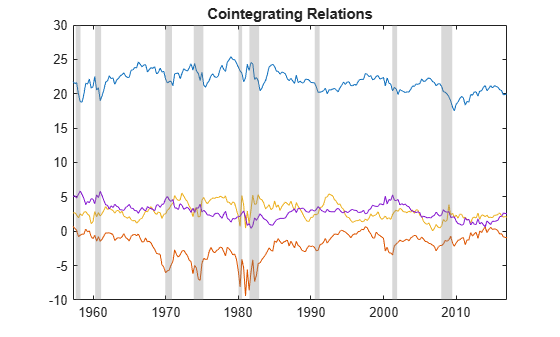The plot suggests that the cointegrating relations are approximately stationary, although periods of volatility and abrupt change appear clustered around economic recessions.

### Impulse Response Analysis

When economic conditions change, especially in response to a policy decision, you can assess the sensitivity of the system using an impulse response analysis.

Compute the impulse response function (IRF) of nominal GDP to a one-standard-deviation shock to each economic variable using the armairf function. By default, armairf displays the orthogonalized impulse response in which the residual covariance matrix is orthogonalized by its Cholesky factorization. The armairf function also supports the generalized impulse response of Pesaran and Shin , but for nominal GDP the results are similar in profile.

The armairf function returns the IRF in a 3-D array. Each page (the third dimension) captures the response of a specific variable, within a given forecast horizon, to a one-standard-deviation shock to all variables in the system. Specifically, element t, j, k is the response of variable k at time t in the forecast horizon, to an innovation shock to variable j at time 0. Columns and pages correspond to the response variables in the data, and t = 0, 1, ... fh, where fh is the forecast horizon length.

Convert the fitted VEC model to its cointegrated VAR representation by using the varm conversion function. Then, compute the IRF for 40 quarters (10 years).

horizon = 40; VAR = varm(Fit); IRF = armairf(VAR.AR, {}, 'InnovCov', VAR.Covariance, 'NumObs', horizon); h = figure; iSeries = 1; % Column 1 is associated with GDP series for i = 1:Mdl.NumSeries subplot(Mdl.NumSeries,1,i) plot(IRF(:,i,iSeries)) title("GDP Impulse Response to " + Data.Properties.VariableNames(i)) end screen = get(h,'Parent'); set(h,'Position',[h.Position(1) screen.MonitorPositions(4)*0.1 h.Position(3) screen.MonitorPositions(4)*0.8]);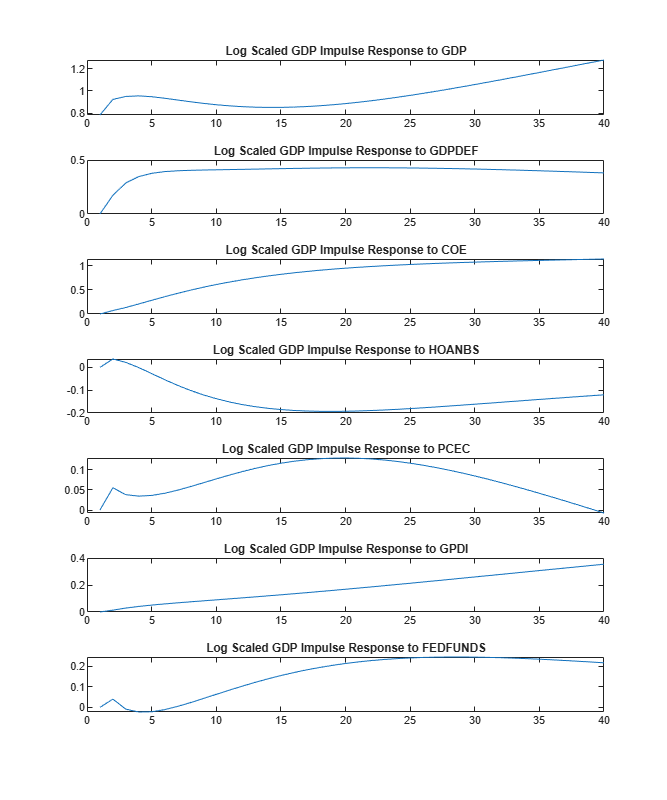Although the preceding plots display the impulse response over a span of 10 years, each response in fact approaches a steady-state asymptotic level indicating a unit root and suggesting that the shocks are persistent and have permanent effects on nominal GDP.

### Compute Out-of-Sample Forecasts and Assess Forecast Accuracy

To test the accuracy of the model, iteratively compute out-of-sample forecasts. Perform an experiment similar to that suggested by Smets and Wouters , pp. 21-22:

1. Estimate the VEC model over the initial 20-year sample period 1957:Q1 - 1976:Q4

2. Forecast the data 1, 2, 3, and 4 quarters into the future.

3. Re-estimate the model over the period 1957:Q1 - 1977:Q1, adding the data of the next quarter to the sample thereby increasing the sample size available for estimation.

4. Repeat the steps 2 and 3, accumulating a time series of forecasts until the end of the sample.

Y = Data; % Work directly with the timetable T0 = datetime(1976,12,31); % Initialize forecast origin (31-Dec-1976 = (1976,12,31) triplet) T = T0; horizon = 4; % Forecast horizon in quarters (4 = 1 year) numForecasts = numel(Y.Time(timerange(T,Y.Time(end),'closed'))) - horizon; yForecast = nan(numForecasts, horizon, Mdl.NumSeries); for t = 1:numForecasts % % Get the end-of-quarter dates for the current forecast origin. % quarterlyDates = timerange(Y.Time(1), T, 'closed'); % % Estimate the VEC model. % Fit = estimate(toFit, Y{quarterlyDates,:}, 'Model', 'H1'); % % Forecast the model at each quarter out to the forecast horizon. % % Store the forecasts for the current origin (T) as a 3-D array in which % the 1st page stores all forecasts for the 1st series (GDP), the 2nd page % stores all forecasts for the 2nd series (GDPDEF), and so forth. This % storage convention facilitates the access of data from the timetable % of forecasts created below. % yForecast(t,:,:) = forecast(Fit, horizon, Y{quarterlyDates,:}); % % Update the forecast origin to include the data of the next quarter. % T = dateshift(T, 'end', 'quarter', 'next'); end 

Compute the forecast origin dates common to all forecasts in the forecast horizon, and the specific quarterly dates at which each of the forecasts applies. Then, create a timetable whose common time stamps (along each row) are the dates of each quarterly forecast origin. At each forecast origin, store the forecasts of each of the 7 series 1, 2, 3, and 4 quarters into the future, as well as the quarterly dates at which each forecast applies. The timetable is formatted this way to facilitate access to the forecasts of a given economic series.

originDates = dateshift(T0, 'end', 'quarter', (0:(numForecasts-1))'); forecastDates = NaT(numForecasts,horizon); for i = 1:horizon forecastDates(:,i) = dateshift(originDates, 'end', 'quarter', i); end Forecast = timetable(forecastDates,'RowTimes',originDates, 'VariableNames', {'Times'}); for i = 1:size(Y,2) Forecast.(Y.Properties.VariableNames{i}) = yForecast(:,:,i); end 

Using the iterative forecasts just obtained, assess the forecast accuracy of real GDP (nominal GDP net of GDP Price Deflator) by plotting its forecasts and true reported values at the forecast horizon (4-quarters-ahead). Also, plot the corresponding forecast errors.

forecastRealGDP = Forecast.GDP(:,4) - Forecast.GDPDEF(:,4); realGDP = Y.GDP(Forecast.Times(:,4)) - Y.GDPDEF(Forecast.Times(:,4)); figure subplot(2,1,1) plot(Forecast.Times(:,4), forecastRealGDP, 'r') hold on plot(Forecast.Times(:,4), realGDP, 'b') title('Real GDP vs. Forecast: 4-Quarters-Ahead') ylabel('USD Billion') recessionplot h = legend('Forecast','Actual','Location','Best'); h.Box = 'off'; subplot(2,1,2) plot(Forecast.Times(:,4), forecastRealGDP - realGDP) title('Real GDP Forecast Error: 4-Quarters-Ahead') ylabel('USD Billion') recessionplot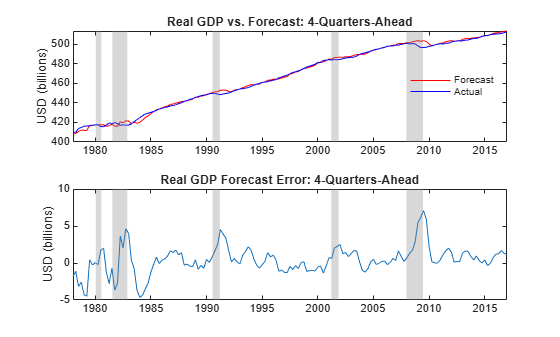The plots illustrate that during and shortly after periods of economic recession the GDP forecasts tend to overestimate the true GDP. In particular, observe the large positive errors in the aftermath of the fiscal crisis of 2008 and into the first half of 2009.

For reference, although the plots do not show the forecast errors of the unconstrained VARX model without exclusion constraints imposed on the constant, short-run, and adjustment parameters, the constrained results in the plots represent approximately a 25 percent reduction in root mean square error (RMSE) of real GDP forecasts.

### Analysis of Fiscal Crisis of 2008: Forecasting Real GDP

Investigate the forecasts in the vicinity of the 2008 fiscal crisis in more detail. Specifically, investigate the forecast performance just prior to the crisis as the economy transitions into recession, and then again just after the crisis as the recovery ensues. For completeness, examine the forecast behavior using the most recent data.

In the style of Smets and Wouters , use a 12-quarter (3-year) forecast horizon. In an attempt to incorporate the forecasts of more than a single series, examine real GDP.

Estimate and forecast real GDP 12 quarters into the future using data to the end of 2007, just prior to the mortgage crisis.

horizon = 12; T = datetime(2007,12,31); % Forecast origin just prior to the crisis (31-Dec-2007 = (2007,12,31) triplet) Fit = estimate(toFit, Y{timerange(Y.Time(1),T,'closed'),:}, 'Model', 'H1'); [yForecast, yMSE] = forecast(Fit, horizon, Y{timerange(Y.Time(1),T,'closed'),:}); sigma = zeros(horizon,1); % Forecast standard errors for i = 1:horizon sigma(i) = sqrt(yMSE{i}(1,1) - 2*yMSE{i}(1,2) + yMSE{i}(2,2)); end forecastDates = dateshift(T, 'end', 'quarter', 1:horizon); figure plot(forecastDates, (yForecast(:,1) - yForecast(:,2)) + sigma, '-.r') hold on plot(forecastDates, yForecast(:,1) - yForecast(:,2), 'r') plot(forecastDates, (yForecast(:,1) - yForecast(:,2)) - sigma, '-.r') plot(forecastDates, Y.GDP(forecastDates) - Y.GDPDEF(forecastDates), 'b') title("Real GDP vs. 12-Q Forecast: Origin = " + string(T)) ylabel('USD Billion') recessionplot h = legend('Forecast +1\sigma', 'Forecast', 'Forecast -1\sigma', 'Actual', 'Location', 'Best'); h.Box = 'off';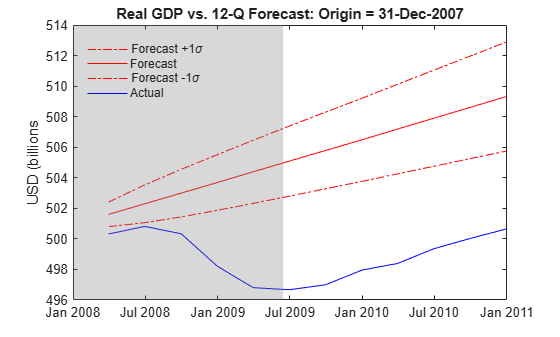The estimated model fails to anticipate the dramatic economic downturn. Given the magnitude of the crisis, the failure to capture the extent of the recession is, perhaps, somewhat unsurprising.

Estimate and forecast real GDP 12 quarters into the future using data up to the middle of 2009, which is just after the mortgage crisis.

T = datetime(2009,6,30); % Forecast origin just after the crisis (30-Jun-2009 = (2009,6,30) triplet) Fit = estimate(toFit, Y{timerange(Y.Time(1),T,'closed'),:}, 'Model', 'H1'); [yForecast, yMSE] = forecast(Fit, horizon, Y{timerange(Y.Time(1),T,'closed'),:}); sigma = zeros(horizon,1); % Forecast standard errors for i = 1:horizon sigma(i) = sqrt(yMSE{i}(1,1) - 2*yMSE{i}(1,2) + yMSE{i}(2,2)); end forecastDates = dateshift(T, 'end', 'quarter', 1:horizon); figure plot(forecastDates, (yForecast(:,1) - yForecast(:,2)) + sigma, '-.r') hold on plot(forecastDates, yForecast(:,1) - yForecast(:,2), 'r') plot(forecastDates, (yForecast(:,1) - yForecast(:,2)) - sigma, '-.r') plot(forecastDates, Y.GDP(forecastDates) - Y.GDPDEF(forecastDates), 'b') title("Real GDP vs. 12-Q Forecast: Origin = " + string(T)) ylabel('USD Billion') h = legend('Forecast +1\sigma', 'Forecast', 'Forecast -1\sigma', 'Actual', 'Location', 'Best'); h.Box = 'off';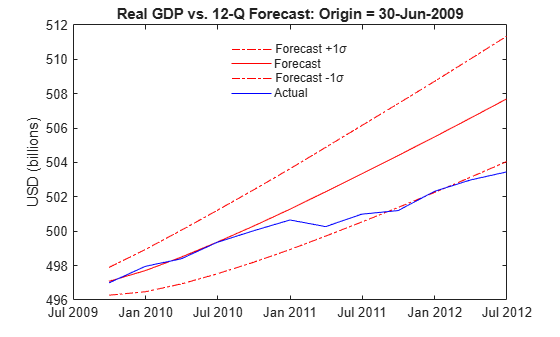Once the recession data is included in the estimation, the forecasts of real GDP agree reasonably well with the true values. Although, beyond the 2-year forecast horizon, the forecasts still present an overly rosy economic recovery.

Finally, incorporate the latest post-crisis data available.

T = dateshift(Data.Time(end), 'end', 'quarter', -horizon); Fit = estimate(toFit, Y{timerange(Y.Time(1),T,'closed'),:}, 'Model', 'H1'); [yForecast, yMSE] = forecast(Fit, horizon, Y{timerange(Y.Time(1),T,'closed'),:}); sigma = zeros(horizon,1); % Forecast standard errors for i = 1:horizon sigma(i) = sqrt(yMSE{i}(1,1) - 2*yMSE{i}(1,2) + yMSE{i}(2,2)); end forecastDates = dateshift(T, 'end', 'quarter', 1:horizon); figure plot(forecastDates, (yForecast(:,1) - yForecast(:,2)) + sigma, '-.r') hold on plot(forecastDates, yForecast(:,1) - yForecast(:,2), 'r') plot(forecastDates, (yForecast(:,1) - yForecast(:,2)) - sigma, '-.r') plot(forecastDates, Y.GDP(forecastDates) - Y.GDPDEF(forecastDates), 'b') title("Real GDP vs. 12-Q Forecast: Origin = " + string(T)) ylabel('USD Billion') h = legend('Forecast +1\sigma', 'Forecast', 'Forecast -1\sigma', 'Actual', 'Location', 'Best'); h.Box = 'off';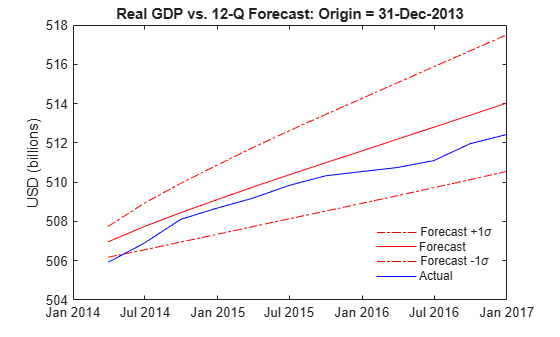Now that the recovery has gained some momentum and stabilized, the 3-year forecasts agree more closely with the true values.

### Sub-Sample Sensitivity: The Great Inflation vs. the Great Moderation

To examine the stability and sensitivity of the analysis, compare the estimation results obtained from two distinct subsamples.

Follow the approach outlined by Smets and Wouters , pp. 28-29, by performing a what-if sensitivity analysis, referred to as a counterfactual experiment. Examine the response of a model fitted to data over one period to shocks derived from a model fitted over another.

Specifically, Smets and Wouters identify the period ending at 1979:Q2 with the appointment of Paul Volker as chairman of the Board of Governors of the Federal Reserve as the Great Inflation, and that starting 1984:Q1 as the Great Moderation. The gap between the Great Inflation and the Great Moderation is, apparently, a transition period and includes the two recessions in the early 1980s.

Estimate the VEC model over the period 1957:Q1 to 1979:Q2, and then again from 1984:Q1 to the latest quarter for which data is available. Although the same pre-estimation model assessment performed for the full sample above could be repeated for each sub-sample, for simplicity retain the same VEC model and subset constraints used previously.

T1 = datetime(1979,6,30); % Last quarter of Great Inflation (30-Jun-1979 = (1979,6,30) triplet) Y1 = Y(timerange(Y.Time(1),T1,'closed'),:); % Great Inflation data [Fit1,~,~,E1] = estimate(toFit, Y1.Variables, 'Model', 'H1'); T2 = datetime(1984,3,31); % First quarter of Great Moderation (31-Mar-1984 = (1984,3,31) triplet) Y2 = Y(timerange(T2,Y.Time(end),'closed'),:); % Great Moderation data Fit2 = estimate(toFit, Y2.Variables, 'Model', 'H1'); 

Filter the inferred residuals obtained from the first sub-sample (the Great Inflation) through the model obtained from the most recent sub-sample (the Great Moderation) using the filter function.

To better compare the filtered responses obtained below to the fitted results of the Great Moderation obtained above, initialize the filter using the first P observations of the Great Moderation sub-sample. In other words, although the residuals inferred from the Great Inflation filter through the fitted model obtained from the Great Moderation, you initialize the filter using data from the beginning of the Great Moderation.

YY = filter(Fit2, E1, 'Y0', Y2{1:Fit2.P,:}, 'Scale', false); Y2 = Y2((Fit2.P + 1):(size(E1,1) + Fit2.P),:); % Extract relevant data for convenient comparison YY = array2timetable(YY, 'RowTimes', Y2.Time, 'VariableNames', Y.Properties.VariableNames); 

Compare real GDP data reported during the Great Moderation to that obtained by filtering the shocks from the Great Inflation through the model fitted to the Great Moderation.

figure plot(YY.Time, Y2.GDP - Y2.GDPDEF, 'b') hold on plot(YY.Time, YY.GDP - YY.GDPDEF, 'r') title('Real GDP Sub-Sample Comparison') ylabel('USD Billion') recessionplot h = legend('Reported Great Moderation Data', 'Filtered Great Inflation Residuals', 'Location', 'Best'); h.Box = 'off';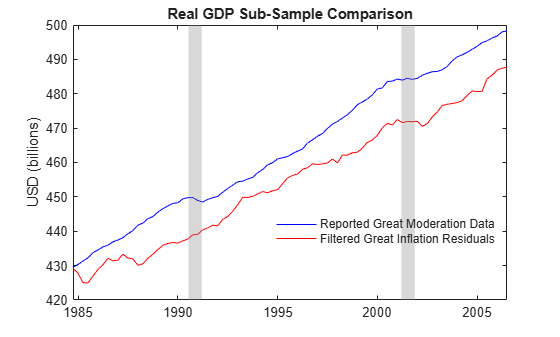The plot suggests that the filtered results are more volatile than the reported Great Moderation data, an observation noted by Smets and Wouters , p. 30.

The filtering just performed filters the residuals obtained from the Great Inflation through the model fitted to the Great Moderation, yet represents only one alternative within a broader historical simulation, filtering, and forecasting framework.

For example, although the analysis to this point has been primarily concerned with GDP, it is interesting to note that the analysis also provides a reasonably accurate representation of the other series as well, with the possible exception of short-term interest rates (FEDFUNDS).

Within the Smets-Wouters model, interest rates are the only series left unadjusted. Moreover, Smets and Wouters include data only through the end of 2004, prior to the mortgage crisis. At the end of 2004 short-term interest rates were already at low levels, yet actually approached zero at the end of 2008 as the fiscal crisis ensued and the recession began. Although the economy recovered, interest rates remained at historically low levels for several years.

Given that VAR and VEC models are linear approximations of the true, yet unknown, vector process whose dependence is captured by a multi-variate Gaussian distribution, it is not surprising to encounter negative interest rates. In fact, although FEDFUNDS rates have never been negative, other short-term interest-bearing accounts, especially in Europe, have been known to provide negative yields.

### Conditional Forecasting & Simulation

Conditional analysis is closely related to stress testing. Specifically, values of a subset of variables are specified in advance, often subjected to adverse conditions or extreme values, in an attempt to assess the effects on the remaining variables during periods of economic crisis.

One approach to modeling economic time series in the presence of near-zero interest rates is to perform a conditional forecast and simulation using the model fitted to the Great Moderation to capture the latest data.

Specifically, the analysis incorporates 10-year economic projections obtained from the Congressional Budget Office (CBO) , which publishes quarterly forecasts for the following subset of the FRED series:

CBO Series Description ------------ ----------------------------------------------------------------- GDP Gross Domestic Product (USD billions) GDPDEF Gross Domestic Product Implicit Price Deflator (Index 2009 = 100) COE Paid Compensation of Employees (USD billions) TB3M 3-Month Treasury Bill Rate (Annualized, Percent)

Although the 3-Month T-Bill and FEDFUNDS rates are not identical, in recent years they are virtually indistinguishable. So, you can use the 3-Month T-Bill rate as a proxy for the FEDFUNDS rate.

To obtain conditional forecasts, create a timetable in which the CBO projections capture the future evolution of known data on which unknown forecasts are conditioned. When formatting the timetable, NaN values indicate unknown (missing) forecasts conditioned on known (non-missing) information.

As for the FRED series, all CBO series except the short-term interest rate are transformed. Transform the series and store them in a new timetable (YF), which captures the subset of series known in advance and serves as the data on which you compute the remaining forecasts.

YF = CBO; % Assign the dates and raw data YF.GDP = 100*log(CBO.GDP); % GDP (output) YF.GDPDEF = 100*log(CBO.GDPDEF); % GDP implicit prices deflator YF.COE = 100*log(CBO.COE); % Compensation of employees (wages) 

Examine the last 4 quarterly CBO projections and observe that hours worked (HOANBS), consumption (PCEC), and investment (GPDI) are missing (NaNs) because the CBO does not publish projections for these series, while GDP, GDP deflator (GDPDEF), compensation (COE), and FEDFUNDS contain the CBO projections.

YF(end-3:end,:) 
ans = 4x7 timetable Time GDP GDPDEF COE HOANBS FEDFUNDS PCEC GPDI ___________ ______ ______ ______ ______ ________ ____ ____ 31-Mar-2027 1023.5 492.1 963.14 NaN 2.8 NaN NaN 30-Jun-2027 1024.4 492.6 964.07 NaN 2.8 NaN NaN 30-Sep-2027 1025.4 493.09 964.99 NaN 2.8 NaN NaN 31-Dec-2027 1026.3 493.59 965.89 NaN 2.8 NaN NaN 

Compare the forecasts of real GDP derived from the CBO projections to those obtained from the conditional forecasts of the VEC model. To forecast nominal GDP conditionally, set the CBO projections of nominal GDP to NaNs to indicate the lack of information regarding nominal GDP. Display the last 4 quarterly projections.

YF.GDP(:) = NaN; YF(end-3:end,:) 
ans = 4x7 timetable Time GDP GDPDEF COE HOANBS FEDFUNDS PCEC GPDI ___________ ___ ______ ______ ______ ________ ____ ____ 31-Mar-2027 NaN 492.1 963.14 NaN 2.8 NaN NaN 30-Jun-2027 NaN 492.6 964.07 NaN 2.8 NaN NaN 30-Sep-2027 NaN 493.09 964.99 NaN 2.8 NaN NaN 31-Dec-2027 NaN 493.59 965.89 NaN 2.8 NaN NaN 

Extract the quarterly CBO projections beyond the date of the most recent FRED data and forecast unknown series conditional on the projections.

The forecast function converts the VEC model to its equivalent state-space representation, and derives the missing forecasts using a Kalman filter. Missing values become the filtered states computed as a minimum mean square error (MMSE) conditional expectation.

forecastQuarters = timerange(Y.Time(end),CBO.Time(end),'openleft'); YF = YF(forecastQuarters,:); yForecast = forecast(Fit2, size(YF,1), Y.Variables, 'YF', YF.Variables); yForecast = array2timetable(yForecast, 'RowTimes', YF.Time, 'VariableNames', Y.Properties.VariableNames); 

Inspect the results to see that the missing GDP, HOANBS, PCEC, and GPDI series are populated with their conditional forecasts, while the GDPDEF, COE, and FEDFUNDS series used for conditioning are unchanged.

yForecast(end-3:end,:) 
ans = 4x7 timetable Time GDP GDPDEF COE HOANBS FEDFUNDS PCEC GPDI ___________ ______ ______ ______ ______ ________ ______ ______ 31-Mar-2027 1024.1 492.1 963.14 488.27 2.8 987.67 837.48 30-Jun-2027 1025 492.6 964.07 488.69 2.8 988.6 838.42 30-Sep-2027 1025.9 493.09 964.99 489.05 2.8 989.5 838.98 31-Dec-2027 1026.7 493.59 965.89 489.4 2.8 990.38 839.51 

Compare real GDP derived from the conditional forecasts to that derived from the CBO projections. Observe that they agree closely.

figure plot(YF.Time, 100*log(CBO.GDP(forecastQuarters)) - YF.GDPDEF, 'b') hold on plot(YF.Time, yForecast.GDP - yForecast.GDPDEF, 'r') title('Real GDP Projection vs. Conditional Forecast') ylabel('USD Billions') h = legend('CBO Real GDP Projection', 'Real GDP Conditional Forecast', 'Location', 'Best'); h.Box = 'off';Conditional analyses is not limited to forecasting alone, and extend to simulation as well. Given a pattern of NaNs and non-NaNs found in YF, you can impute unknown values by sampling from a conditional, multivariate Gaussian distribution.

Simulate 1000 conditional sample paths with the simulate function.

rng default YY = simulate(Fit2, size(YF,1), 'Y0', Y.Variables, 'YF', YF.Variables, 'NumPaths', 1000); 

Plot, for example, the first 10 sample paths of real GDP.

figure for i = 1:10 plot(YF.Time, YY(:,1,i) - YY(:,2,i)) hold on end title('Real GDP Sample Paths') ylabel('USD Billion')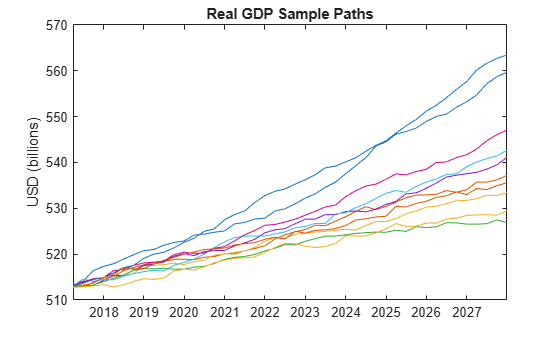The plot suggests that the distribution of real GDP varies significantly as a function of time, and so another metric of interest is the distribution of real GDP.

Plot the empirical probability density function (PDF) of real GDP 5 years (20 quarters) into the future.

YY = permute(squeeze(YY(20,:,:)), [2 1]); figure histogram(YY(:,1) - YY(:,2), 'Normalization', 'pdf') xlabel('Real GDP (USD Billion)') title('5-Year-Ahead Density of Real GDP')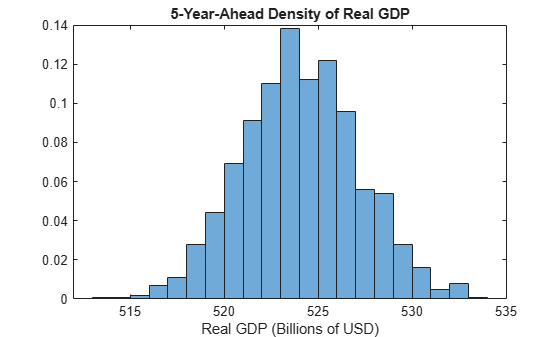Congressional Budget Office, Budget and Economic Data, 10-Year Economic Projections, https://www.cbo.gov/about/products/budget-economic-data#4.

 Del Negro, M., Schorfheide, F., Smets, F. and Wouters, R. "On the Fit of New Keynesian Models." Journal of Business & Economic Statistics. Vol. 25, No. 2, 2007, pp. 123–162.

 Hamilton, James D. Time Series Analysis. Princeton, NJ: Princeton University Press, 1994.

 Johansen, S. Likelihood-Based Inference in Cointegrated Vector Autoregressive Models. Oxford: Oxford University Press, 1995.

 Juselius, K. The Cointegrated VAR Model. Oxford: Oxford University Press, 2006.

 Kimball, M. "The Quantitative Analytics of the Basic Neomonetarist Model." Journal of Money, Credit, and Banking, Part 2: Liquidity, Monetary Policy, and Financial Intermediation. Vol. 27, No. 4, 1995, pp. 1241–1277.

 Lütkepohl, Helmut, and Markus Krätzig, editors. Applied Time Series Econometrics. 1st ed. Cambridge University Press, 2004. https://doi.org/10.1017/CBO9780511606885.

 Lütkepohl, Helmut. New Introduction to Multiple Time Series Analysis. New York, NY: Springer-Verlag, 2007.

 National Bureau of Economic Research (NBER), Business Cycle Expansions and Contractions, https://www.nber.org/research/data/us-business-cycle-expansions-and-contractions.

 Pesaran, H. H., and Y. Shin. "Generalized Impulse Response Analysis in Linear Multivariate Models." Economic Letters. Vol. 58, 1998, pp. 17–29.

 Smets, F. and Wouters, R. "An Estimated Stochastic Dynamic General Equilibrium Model of the Euro Area." European Central Bank, Working Paper Series. No. 171, 2002.

 Smets, F. and Wouters, R. "Comparing Shocks and Frictions in US and Euro Area Business Cycles: A Bayesian DSGE Approach." European Central Bank, Working Paper Series. No. 391, 2004.

 Smets, F. and Wouters, R. "Shocks and Frictions in US Business Cycles: A Bayesian DSGE Approach." European Central Bank, Working Paper Series. No. 722, 2007.

 U.S. Federal Reserve Economic Data (FRED), Federal Reserve Bank of St. Louis, https://fred.stlouisfed.org/.